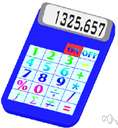# arithmetic mean

Also found in: Thesaurus, Medical, Legal, Financial, Acronyms, Encyclopedia, Wikipedia.

## ar·ith·met·ic mean

(ăr′ĭth-mĕt′ĭk)
n.
The value obtained by dividing the sum of a set of quantities by the number of quantities in the set. Also called average.

## arithmetic mean

n
(Mathematics) an average value of a set of integers, terms, or quantities, expressed as their sum divided by their number: the arithmetic mean of 3, 4, and 8 is 5. Often shortened to: mean Also called: average Compare geometric mean
Collins English Dictionary – Complete and Unabridged, 12th Edition 2014 © HarperCollins Publishers 1991, 1994, 1998, 2000, 2003, 2006, 2007, 2009, 2011, 2014

## ar′ithmet′ic mean′

n.
the mean obtained by adding several quantities together and dividing the sum by the number of quantities: The arithmetic mean of 1, 5, 2, and 8 is 4. Also called average.

## ar·ith·met·ic mean

(ăr′ĭth-mĕt′ĭk)
The value obtained by dividing the sum of a set of quantities by the number of quantities in the set. For example, if there are three test scores 70, 83, and 90, the arithmetic mean of the scores is their sum (243) divided by the number of scores (3), or 81. See more at mean. Compare average, median, mode.
ThesaurusAntonymsRelated WordsSynonymsLegend:
 Noun 1arithmetic mean - the sum of the values of a random variable divided by the number of valuesstatistics - a branch of applied mathematics concerned with the collection and interpretation of quantitative data and the use of probability theory to estimate population parametersmean, mean value - an average of n numbers computed by adding some function of the numbers and dividing by some function of n
Based on WordNet 3.0, Farlex clipart collection. © 2003-2012 Princeton University, Farlex Inc.
Translations
aritmetický průměr
aritmeettinen keskiarvokeskiarvo
aritmetička sredina
media aritmetica

średnia arytmetyczna

## arithmetic mean

Collins German Dictionary – Complete and Unabridged 7th Edition 2005. © William Collins Sons & Co. Ltd. 1980 © HarperCollins Publishers 1991, 1997, 1999, 2004, 2005, 2007
References in periodicals archive ?
The table shows that the 'Information Quality' dimension scored an arithmetic mean of (3.60) and a standard deviation of (0.67).
(1.) The arithmetic mean is a measure of central tendency produced by dividing the sum of the prices of all items by the total number of items.
An Internet search of "wet-bulb temperature and arithmetic mean" in January 2017 revealed only a few relevant links; one such link, to a nineteenth-century thermodynamics textbook, includes the statement (without derivation) that "(a)t 53[degrees]F [11.67[degrees]C] the reading of the wet-bulb thermometer is the arithmetic mean between the dew-point and the temperature of the air...
The experimental (E) group showed statistically significant improvement of the results in almost all anthropometric variables by comparing the arithmetic mean of the initial measurement with the final measurement.
Harmonic mean is the reciprocal of the arithmetic mean of reciprocal which is a conservative average to be used to provide for aggregation lying between the max and min operators and is widely used as a tool to aggregate central tendency data which is usually expressed in exact numerical values .
Counters may also be used to prove some simple conjectures about the arithmetic mean. For example, it may be readily observed that (n + 1) is the arithmetic mean of n, (n + 1) and (n + 2).
The CPI uses a hybrid of geometric and arithmetic mean calculation, depending on whether "lower level" or "upper level" indexes are being constructed.
Integrates with 38 out of 46 third party software analysed while the category's arithmetic mean is 19/46.
[A.sub.exp] and [A.sub.p] are called multiplicative arithmetic mean and p-arithmetic mean (as usually known p-mean), respectively.
Then, calculate the arithmetic mean (additive) or geometric mean (multiplicative) for the elements [A.sub.ij] in these positions.
"The substantial reduction in the safe harbor MADL for lead, coupled with OEHHA's draft proposals to require the arithmetic mean for determining average consumption and to prohibit averaging of concentration levels across 'lots,' will further arm private enforcers with even more opportunities to challenge businesses under Prop 65."

Site: Follow: Share:
Open / Close